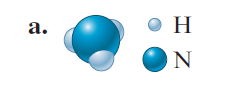# Problem: What amount (moles) of compound is present in 1.00 g of the compound below?

###### FREE Expert Solution

For this problem, we're being asked what amount (moles) of compound is present in 1.00 g

The molecule appears to be: 3 H + 1 N = NH3

81% (327 ratings)###### Problem Details

What amount (moles) of compound is present in 1.00 g of the compound below?Frequently Asked Questions

What scientific concept do you need to know in order to solve this problem?

Our tutors have indicated that to solve this problem you will need to apply the Mole Concept concept. You can view video lessons to learn Mole Concept. Or if you need more Mole Concept practice, you can also practice Mole Concept practice problems.

What professor is this problem relevant for?

Based on our data, we think this problem is relevant for Professor Hammerich's class at UIC.

What textbook is this problem found in?

Our data indicates that this problem or a close variation was asked in Chemistry: An Atoms First Approach - Zumdahl Atoms 1st 2nd Edition. You can also practice Chemistry: An Atoms First Approach - Zumdahl Atoms 1st 2nd Edition practice problems.